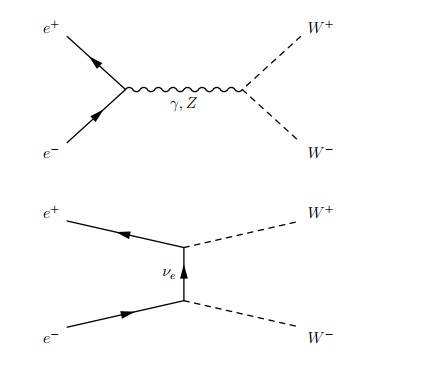# Feynman diagram

## Homework Statement

Hi there,
I am a very beginner in particle physics and now I'm struggling with this question.
Draw the lowest-order Feynman diagrams for e+e- --> W+W- process

## The Attempt at a Solution

There are 3 answers as follows:Q1: 1st pic: Why did the gamma and Z take place in s -channel but not t -channel?
Q2: 2nd pic: Is there a reason why (e-) neutrino is the force carrier? Couldn't it be other entities like gamma or
Z or sth else?

Thanks

#### Attachments

vela
Staff Emeritus
Homework Helper
Q1: You need the electrons going in and the Ws coming out. You can only do that with the s-channel with a photon or Z. To have the t-channel, you'd need to turn the diagram sideways, which would correspond to, for example, an electron and W+ interacting to produce the positron and W-.

Q2: There are only certain allowed vertices. You can't have an electron, W, and photon at one vertex. That would violate conservation of lepton number, for one thing.

Do you have a textbook? There's probably a page showing diagrams of the allowed vertices.

Q1: You need the electrons going in and the Ws coming out. You can only do that with the s-channel with a photon or Z. To have the t-channel, you'd need to turn the diagram sideways, which would correspond to, for example, an electron and W+ interacting to produce the positron and W-.

Q2: There are only certain allowed vertices. You can't have an electron, W, and photon at one vertex. That would violate conservation of lepton number, for one thing.

Do you have a textbook? There's probably a page showing diagrams of the allowed vertices.

I am just following my lecturer's note and he didn't say when we are going to use s or t-channel.I apologize but I'm really confused since this is my first course in particle physics, and I have no previous knowledge in QED so that a lot of course notes online are too difficult for me...I'd like to know, if time is flowing from left to right, in both s and t channels? (1st and 2nd pic)
To me, the only difference between 1st and 2nd pic is that:
1) e- doesn't physically touch e+ in 2nd pic
2) The exchange particle is different , but I don't know why the force carrier is a neutrino ( It could also be a neutrino in 1st pic)
3) You mentioned the conservation of lepton number, so according to 2nd diagram, we now have ve,e- and W- at the lower vertex. So Le = 1 + 1 = 2
At the upper vertex,Le = 1 - 1 = 0 ?
Let say if ve is replaced by a photon, then L is still different at both vertices ( -1 and 1) ?

Sorry...Please helpvela
Staff Emeritus
Homework Helper
I'd like to know, if time is flowing from left to right, in both s and t channels? (1st and 2nd pic)
Yes

To me, the only difference between 1st and 2nd pic is that:
1) e- doesn't physically touch e+ in 2nd pic
2) The exchange particle is different, but I don't know why the force carrier is a neutrino ( It could also be a neutrino in 1st pic)
3) You mentioned the conservation of lepton number, so according to 2nd diagram, we now have ve,e- and W- at the lower vertex. So Le = 1 + 1 = 2
At the upper vertex,Le = 1 - 1 = 0 ?
Let say if ve is replaced by a photon, then L is still different at both vertices ( -1 and 1) ?

Sorry...Please helpLike I said in my first reply, there are only certain allowed vertices. Unless you recognize that fact, these diagrams are going to seem random to you.

Take a look at http://www.hep.phy.cam.ac.uk/~chpotter/particleandnuclearphysics/Lecture_05_FeynmanDiagrams.pdf. It may help you make sense of what's going on.

•davon806